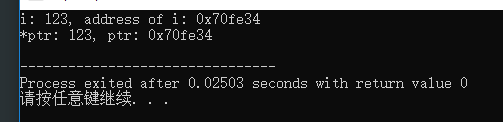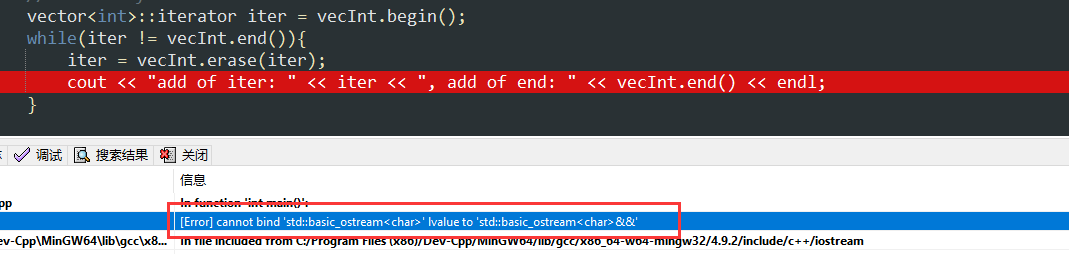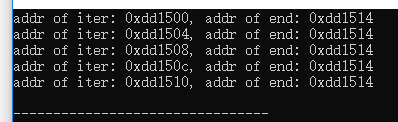• 在使用vector时，使用*iterator可以读出迭代器指向vector中的值，那如果想读出iterator的地址，该怎么表示呢？ 思考 对于常规的指针而言，可以用int *ptr来表示一个指向整型变量的指针，*ptr即指向整型变量的值，ptr...
在使用vector时，使用*iterator可以读出迭代器指向vector中的值，那如果想读出iterator的地址，该怎么表示呢？
思考
对于常规的指针而言，可以用int *ptr来表示一个指向整型变量的指针，*ptr即指向整型变量的值，ptr即地址 代码如下
#include <iostream>
using namespace std;

int main(){
int i = 123;
int *ptr;
ptr = &i;

cout << "i: " << i << ", address of i: " << &i << endl;
cout << "*ptr: " << *ptr << ", ptr: " << ptr << endl;

return 0;
}

打印的结果和预期是一样的尝试去获取iterator的地址
Demo
#include <iostream>
#include <vector>
using namespace std;

int main(){
vector<int> vecInt;
for(int i = 10; i != 15; i++){
vecInt.push_back(i);
}
//show all elements
cout << "show all elements: " << endl;
for(int i : vecInt){
cout << i << " ";
}

vector<int>::iterator iter = vecInt.begin();
while(iter != vecInt.end()){
/*

want to get the address of iterator

cout << "address of iter: " << ??? << ", address of end: " << ??? << endl;

*/
iter++;
}

return 0;
}

在代码中，遍历这个vector，并打印出每个iterator的地址，如果使用和指针类似的写法，如下代码：
vector<int>::iterator iter = vecInt.begin();
while(iter != vecInt.end()){
iter = vecInt.erase(iter);
cout << "addr of iter: " << iter << ", addr of end: " << vecInt.end() << endl;
}

编译器会报错：[Error] cannot bind ‘std::basic_ostream’ lvalue to ‘std::basic_ostream&&’那么应该如何获取呢？

获取iterator的地址
一般来说，迭代器是个数据结构，会将真实的数据地址保存在某个内部的成员变量中，并重载各种运算符（比如解引用运算符*）。
#include <iostream>
#include <vector>
using namespace std;

int main(){
vector<int> vecInt;
for(int i = 10; i != 15; i++){
vecInt.push_back(i);
}

vector<int>::iterator iter = vecInt.begin();
while(iter != vecInt.end()){
cout << "addr of iter: " << &*iter << ", addr of end: " << &*vecInt.end() << endl;
iter++;
}

return 0;
}

通过使用 &*iter来获取迭代器的地址展开全文iterator &* addressof
转换主要是使用stl中的advance和distance函数来进行的， advance是将iterator移动指定个元素，distance是计算两个iterator直接的距离。
distance计算第一个参数到第二个参数之间的距离。如果第二个参数的顺序在第一个参数前面的话,函数是会返回负值的；如果迭代器不在一个容器内,程序会抛出异常。
#include #include #include using namespace std; int main () {   list mylist;   for (int i=0; i<10; i++)   mylist.push_back (i*10.0);
list::iterator first = mylist.begin();   list::iterator last = mylist.end();   advance(first, 1);  cout << "The distance is: " << distance(first,last) << endl;   return 0; }
展开全文• 本篇主要介绍了关于二维vector在使用下标填入或者迭代器时会出现的一种bug，描述了错误写法并且给出了正确的写法。
关于二维vector初始化

文章目录
关于二维vector初始化1.本篇主题以及问题描述2.错误案例3.正确做法

1.本篇主题以及问题描述
二维vector如果想使用下表或者迭代器，使用前必须要指定vector的size，否则会报错。
#include<vector>
// times[i] = (ui, vi, wi)，其中 ui 是源节点，vi 是目标节点， wi 是一个信号从源节点传递到目标节点的时间。
vector<vector<int>>times = [[2,1,1],[2,3,1],[3,4,1]];
// 下面需要根据times构建邻接图

2.错误案例
vector<vector<pair<int, int>>> graph; // 不初始化大小，直接报错
for(auto time : times){ //为了不浪费空间，对每个node-1以便从0开始
graph[time - 1].emplace_back(time-1, time);
}

运行结果（报错）
Line 1034: Char 34: runtime error: applying non-zero offset 24 to null pointer (stl_vector.h)
SUMMARY: UndefinedBehaviorSanitizer: undefined-behavior /usr/bin/../lib/gcc/x86_64-linux-gnu/9/../../../../include/c++/9/bits/stl_vector.h:1043:34

3.正确做法
vector<vector<pair<int, int>>> graph(4); // 为了能够使用下标，我们进行初始化大小
for(auto time : times){ //为了不浪费空间，对每个node-1以便从0开始
graph[time - 1].emplace_back(time-1, time);
}

展开全文c++ stl
• //查找元素，返回对应下标 int findElement(vector<char> v, char key){ int len = v.size(); for(int i=0; i; i++){ if(v.at(i) == key){ return i; } } return -1; } //vector::iterator findElement2...
#include <iostream>
#include <iomanip>
#include <string.h>
#include <cmath>
#include <algorithm>//算法头文件
#include <fstream>
#include <cstdlib>
#include <vector>
#include <sstream>
using namespace std;

//查找元素，返回对应下标
int findElement(vector<char> v, char key){
int len = v.size();
for(int i=0; i<len; i++){
if(v.at(i) == key){
return i;
}
}
return -1;
}
//vector<char>::iterator findElement2(vector<char> v, char key){
//	return find(v.begin(), v.end(), key);
//}
int findElement2(vector<char> v, char key){
vector<char>::iterator it;	//此迭代器用来判断查找元素在不在容器内
it = find(v.begin(), v.end(), key);
if(it != v.end()){
//distance计算第一个参数到第二个参数之间的距离。如果第二个参数的顺序在第一个参数前面的话,函数是会返回负值的；如果迭代器不在一个容器内,程序会抛出异常。
return distance(v.begin(),it);
}
return -1;
}

int main(){

vector<char> v;
v.push_back('a');
v.push_back('b');
v.push_back('c');
v.push_back('a');
v.push_back('a');

cout<<findElement(v,'c')<<endl;

cout<<findElement2(v,'c')<<endl;

return 0;
}


展开全文• 两种写法： int index = &... vector<int> nums{10, 1, 2, 4, 7, 2}; auto it = nums.begin(); it += 4; int index = &*it - &nums; //index 即为it对应元素的下标 int inde.c++
• 迭代器（iterator）方式 vs 下标(operator[])方式 vector遍历iterator C++容器
• 这样定义的一个是迭代器 begin(),end(),返回的是指向容器首元素和末尾元素后一个的两个指针。 #include "mylib7.h" #include <fstream> vector<int>::iterator finds(vector<int>::iterator ...c++ c语言
• #include&lt;iostream&gt;#include&lt;vector&gt;#include&lt;string&gt;using namespace std;int main(){ string s("this is a test"... //这里没用迭代器，直接下标操作  ...
• 这篇博客，通过简单实现vector中的迭代器，了解迭代器的机制 一、迭代器的设计思想： 为什么要有迭代器？ 答：迭代器模式（Iterator ）：提供一种方法顺序访问聚合对象中的每个元素，而不是暴露其内部表示。 要...
• ## vector迭代器的使用

千次阅读 2018-07-30 11:00:44
c++更趋向于使用迭代器而不是下标操作，因为标准库为每一种标准容器（如vector）定义了一种迭代器类型，而只有少数容器（如vector）支持下标操作访问容器元素。 vector使用示例： #include&amp;amp;amp;lt;...
• 大家知道vector容器与数组的不同之处在于，vector可以自动扩容它的容量，是一个动态的数组，而我们的普通数组的容量时固定死的。当vector内元素的数量等于vector容量的时候，再向vector中插入数据，vector会自动申请...
• 原文地址：vector迭代器和数组与指针作者：潴O潴C++基础知识（二）  ——vector类型与迭代器和数组类型与指针 vector类型与数组类型比较 vector类型长度是动态的，可以根据需要动态的调用push_back函数添加新的...
• #include <vector> #include <iostream> using namespace std; int main() ... vector<...//使用类模板制作一个容器... //创建v容器的迭代器对象,迭代器是用来定位容器中某个元素 v.push_back(2); //添.
• 注意：for循环中使用“！=”来代替“&...1、vector可以保存系统的数据类型也可以保存自定义对象类型：vector&lt;int&gt; ivec;//ivec holds object of int .vector&lt;my_type&gt; m...C iterator
• 1、C++为每种容器都定义了迭代器（iterator），只有少数的容器支持下标操作，现在倾向于使用迭代器操作容器。 2、迭代器类似于指针，可以进行引领操作*，自增操作，算数操作，逻辑操作，等等。 3、迭代器的...C++ 容器
• Vector.erase(Vector.begin() + 下标); 例如： vector<int> v; v.erase(v.begiin()+5); 则删除容器中下标为5的元素。c语言 iterator
• C++（STL）容器处理大量数据时的遍历操作，代码效率情况如何，今天做一个系统的效能测试，对比写不同...我们选用最简单的vector容器作为测试目标。先看下测试代码： void main() { vector<byte> tagv; ...c++ STL 标准模板库 访问效率
• vector的二维初始化： vector<int> array2(4, 50);//4个元素每个值为50 数组初始化vector int array[] = { 1,2,5,9,33,2,5,5,4,2 }; vector<int>p(array,array+sizeof(array)/sizeof(array); 增加...c++
•  ——vector类型与迭代器和数组类型与指针 vector类型与数组类型比较 vector类型长度是动态的，可以根据需要动态的调用push_back函数添加新的元素，而数组的长度是固定的；数组没有获取数组大小的函数，而...
• STL内部实现时，首先分配一个非常大的内存空间预备进行存储，即capacituy（）函数返回的大小，当超过此分配的空间时再整体重新放分配一块内存存储，这给人以vector可以不指定vector即一个连续内存的大小的感觉。...list erase C
• 今天验证下在　c++ vector 下标访问及迭代器访问的效率问题。100W 数据存放到　vector 里。便利访问，以为打印的效率很低，所以不打印，只是访问下就　ok。 首先用迭代器的方式访问了一下。 平均时间: real 0m0....c++ it++
• vector是一个类模板 模板本身不是类或函数。 vector的长度类型是跟string相似：string::size_type, vector<类型>::size_type vector<int> v1; // 保持int类型 vector<string> V2; // vector<...
• 也就是意味着可以采用下标vector的元素进行访问，和数组一样高效。但是又不像数组，它的大小是可以动态改变的，而且它的大小会被容器自 动处理。 本质讲，vector使用动态分配数组来存储它的元素。当新元素插入时候...
• #include #include #include #include #include #include #include #include #include #include #include using namespace std; map m; map :: iterator it, firs, e;...vector v; int a; int mai
• ## vector容器与iterator迭代器

万次阅读 多人点赞 2012-08-02 16:28:15
vector容器 vector是同一种类型的对象的集合，每个对象都有一个对应的整数索引值。和string对象一样，标准库负责管理存储元素的相关内存。我们把vector称为容器，是因为它可以包含其他对象。一个容器中的所有对象都...iterator string 测试 each
• 初始化： ...注意：不能通过下标访问，因为刚开始里面没有元素 v.push_back(1); printf("%d",v);//正确 vector<int> v(100,0)); printf("%d",v);//正确 // vector<vector<int&g...